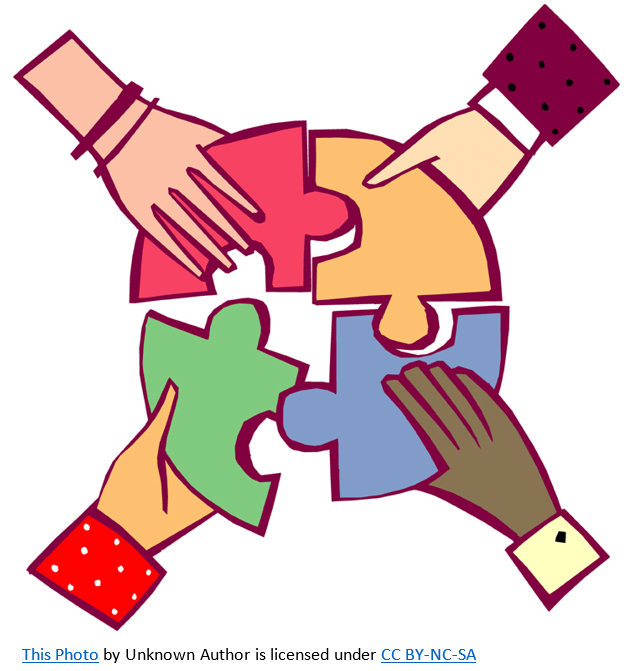# GEOM 3 | Lesson 2 | Making ConnectionsExamine the triangle below.  If you reflect the triangle over the $$y$$-axis.  What is true about the image?    How about over the $$x$$-axis?  Now draw the triangle with coordinates $$(-3, 0), (0, 4)$$ and $$(3,0)$$.  Reflect it over both axes.  What do you notice?Now draw the triangle with coordinates $$(-3, 0), (0, 4), \text{ and } (3,0)$$.  What type of triangle is formed?   Notice that the y-axis is the axis of symmetry with respect to that triangle.  What conclusions can you draw?

Provide two real world examples where congruent triangles are needed to create symmetry or congruence.